# Reference

Calculated items take a set of variables, applies an aggregation to each of the input variables to align them in time and finally applies a formula that returns a single value for each timestamp. If we break this down, we can start defining what a calculated item will look like.

### 🚧Requirements

To create a new calculated item, you will need to be an administrator of your organization.

A calculated item contains three basic components, a set of variables, a formula and an aggregation setting.

# Variables

The input to a calculated item is its variable(s), where each variable is an existing item in Clarify., these provide us with the data to perform the calculation on. When we choose the items we want to use, each one gets assigned a variable name that we can use when referring to it in our formula.

# Aggregation

The aggregation for a calculated item is applied to all the variables, and is defined by a time period, and an aggregation method. Examples of this could be `sum(1 hour)` or `average(15 minute).

When we perform our calculation, we will take the value of each variable, at each available timestamp, as input. Since items don't necessarily have timestamps that are exactly the same, let's consider what would happen both when they do and when they don't.

Timestamps exactly overlap

For items where the timestamps perfectly overlap, there is no need to aggregate the data before performing calculations. We simply want to take the value of each item, at each timestamp, and perform our calculation.

### 🚧What to do when you don't need aggregation

When defining calculated items in Clarify, you need to specify an aggregation. But what should we do in cases where we know that the timestamps align, and we want to preserve the original resolution of the data? In these cases, we can simply set the aggregation to be a higher resolution than the original data.

As an example, if you want to add together two items where values are stored once per hour, setting the aggregation time to once per minute will result in keeping the original resolution.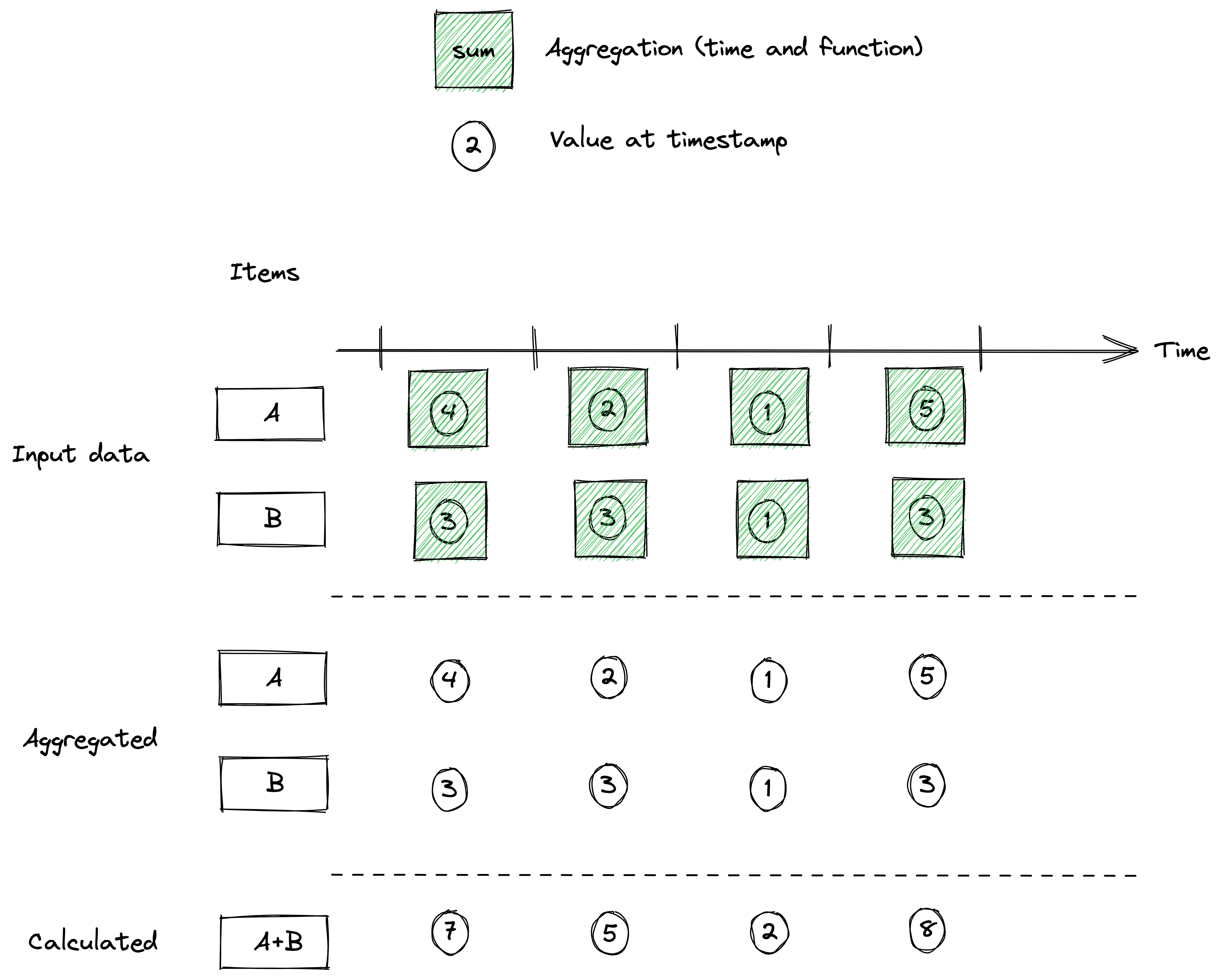Timestamps don't overlap

If the timestamps don't overlap we can use aggregation to re-align them in a way that makes us able to perform the calculation we want.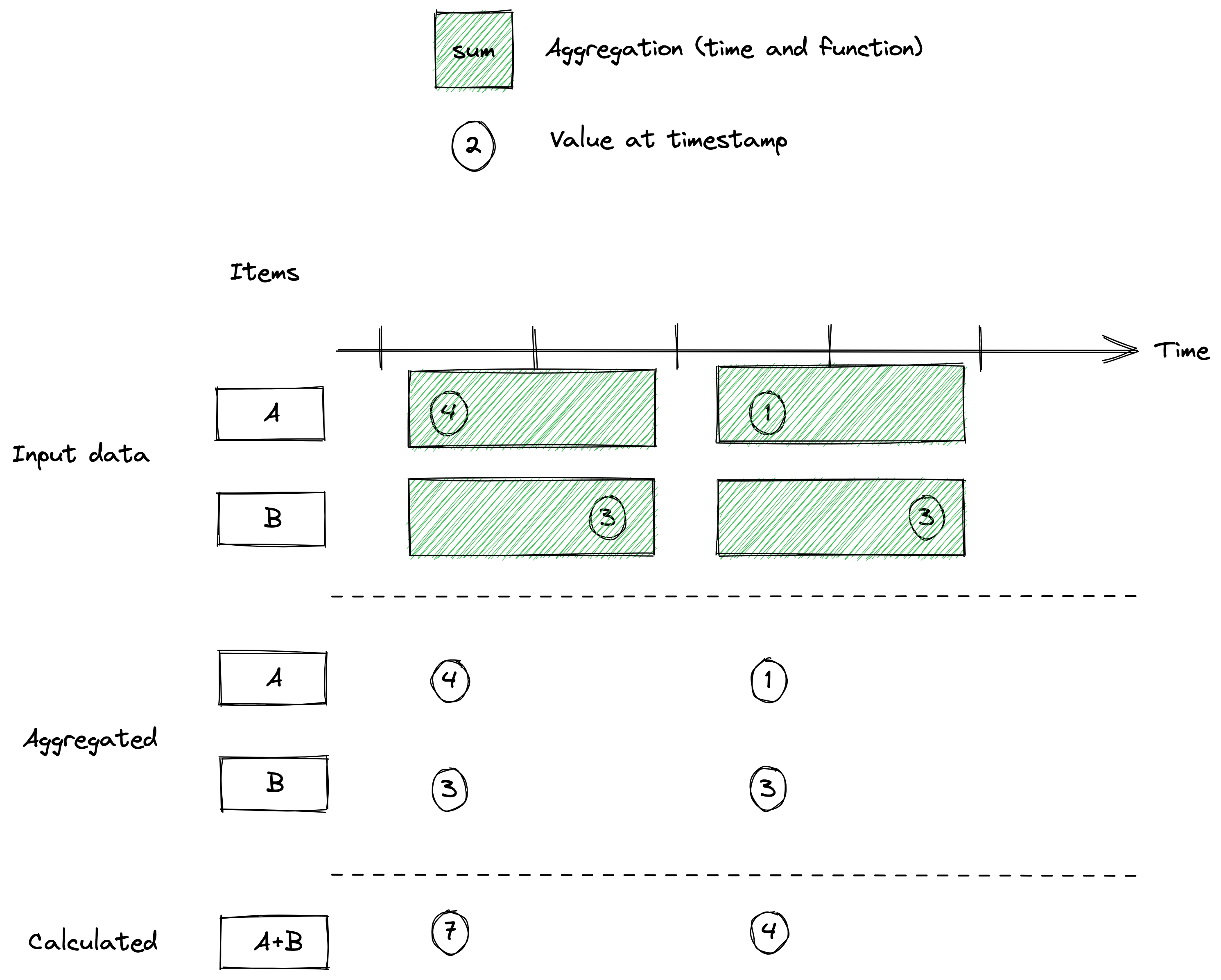Let's also consider one last case that we can use aggregation time for, which is downsampling our data prior to performing calculations. This happens if we set the aggregation time high enough that it covers more than one sample for each variable. In that case, multiple samples for each variable will be aggregated together, and the result of that will be used for the calculation.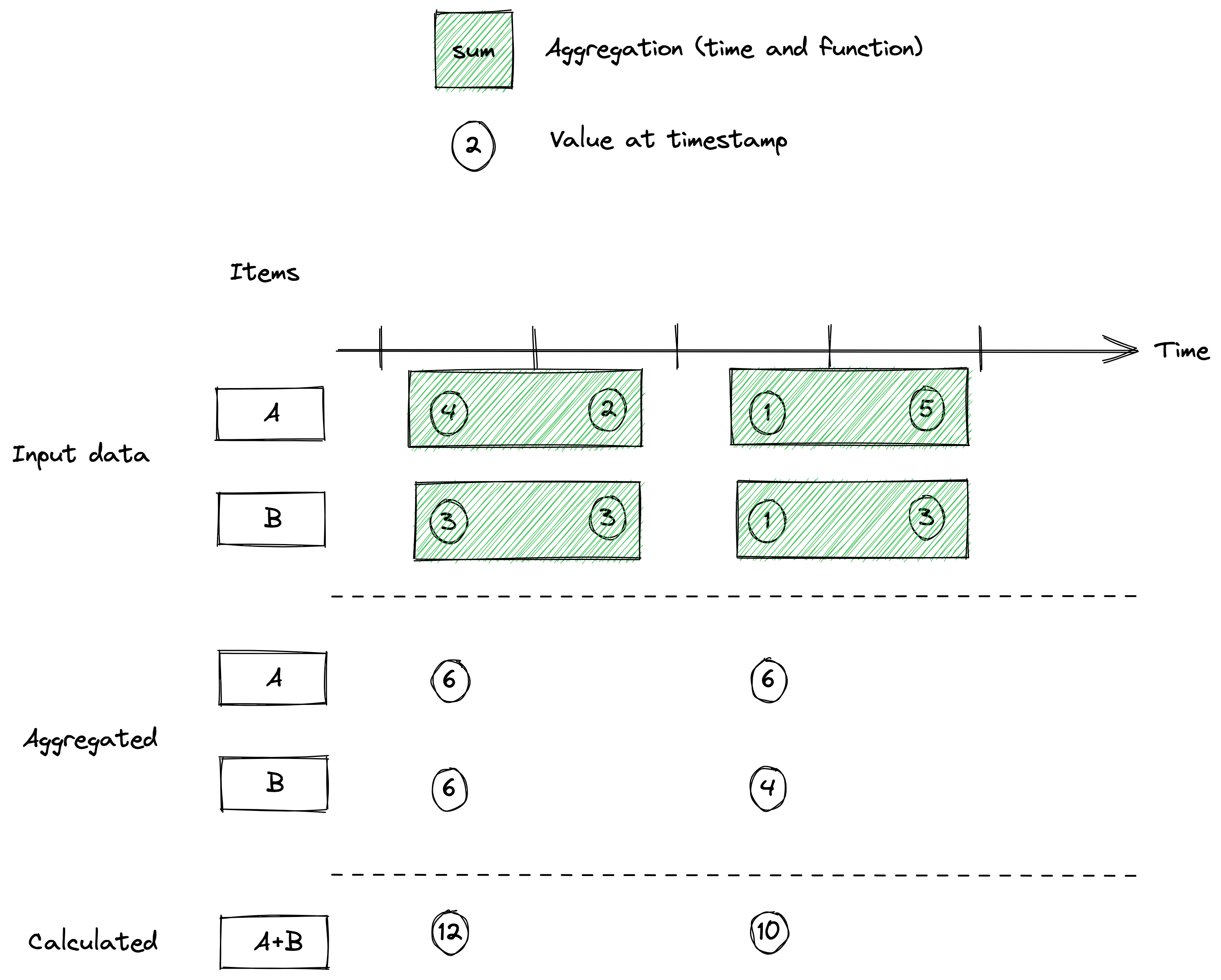# Formula

The formula tells Clarify what to do with the values for each time stamp of the variables after the aggregation step. Calculated items currently support the following operators:

• `+`
• `-`
• `*`
• `/`

By default, division and multiplication is evaluated before addition and subtraction. To explicitly control the order of evaluation, you use parenthesis.

Example formulas

• Add together three variables `a + b + c`.
• Calculate average of two variables `(a + b) / 2`
• Multiply by a constant `a * 10`
• Calculate a complicated KPI `(a * 1.42) + (b / (d * 2))`

# Omitted values

Even with aggregation there will be cases where not all variables in a calculation will have values at every timestamp. In these situations, it's important to understand what Clarify will do with the output values.

Clarify handles omitted values differently based on the operation taking place, in cases where we are using `+` for example, omitted values will be treated as 0, meaning thew calculation will still take place. In cases where we are performing calculations with `*` or `/` however, Clarify will not perform a calculation if a value is missing.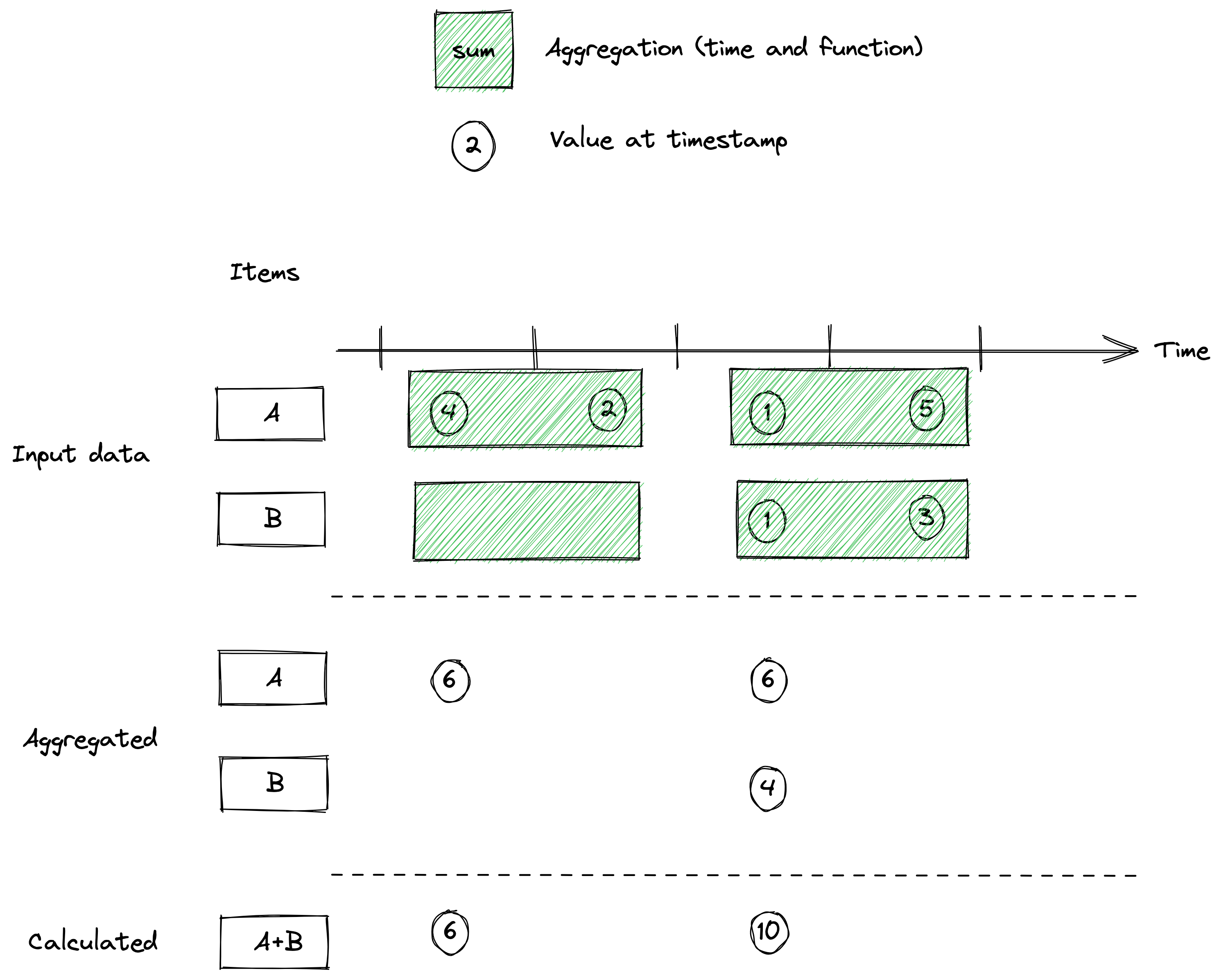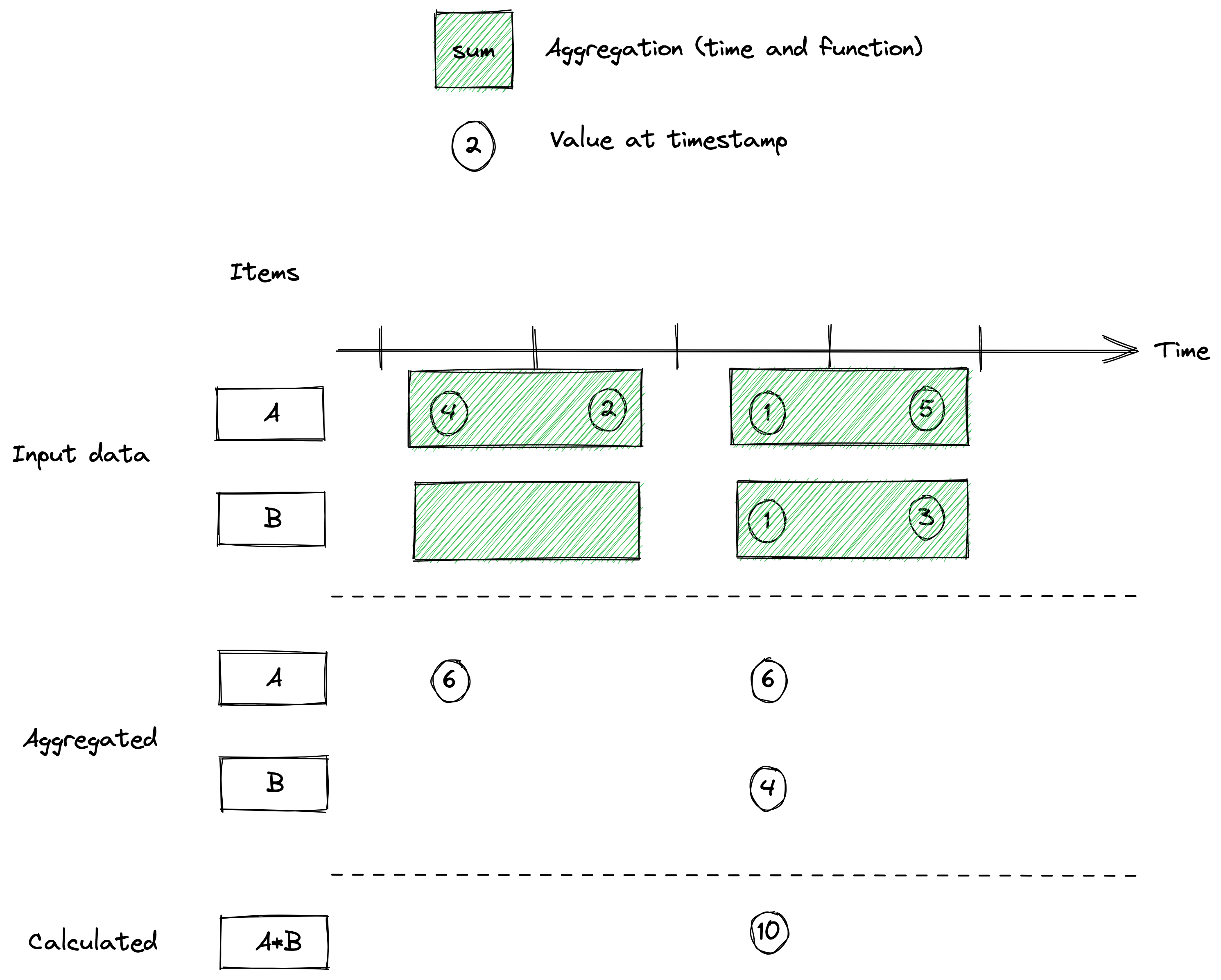Below is a list of the potential situations that can arise, as well as the result that will be returned.

• `0 / 0 = omitted`
• `omitted / X = omitted`
• `X / 0 = omitted`
• `X / omitted = omitted`
• `X * omitted = omitted`
• `X + omitted = X`
• `X - omitted = X`
• `omitted + X = X`
• `omitted - X = -X`